Worksheets and No Prep Teaching Resources
Math Worksheets

# Area and Perimeter Worksheets

Getting students' heads around the ideas of perimeter and area can be a challenge, but not if students have these amazing worksheets. Graphic representations of these concepts, exciting variations, and compare and contrast exercises make these worksheets unique. Worksheets are also customizable so each student, grades one through six, gets individual attention to systematically increase skills while they have a great time.

Fewer Problems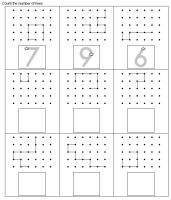Line length of 3 to 9 - first row answers given Line length of 3 to 9 Line length of 10 to 19 - first row answers given Line length of 10 to 19

More Problems Per Page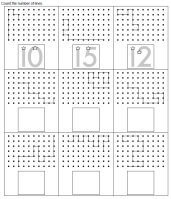Line length of 3 to 9 - first row answers given Line length of 3 to 9 Line length of 10 to 19 - first row answers given Line length of 10 to 19

Connect the Line from A to B - Draw the Path to the Given Line Length (Grades 3-5)
Easier - Starting and Ending Part of Line Given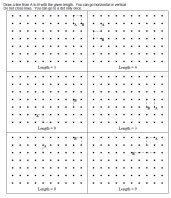Draw the line length of 3 to 9 - first row answers given Draw the line length of 3 to 9 Draw the line length of 10 to 19 - first row answers given Draw the line length of 10 to 19

Draw the Line from A to B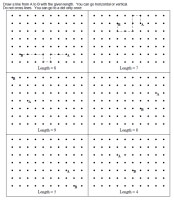Draw the line length of 3 to 9 - first row answers given Draw the line length of 3 to 9 Draw the line length of 10 to 19 - first row answers given Draw the line length of 10 to 19

Easier - Dot Grid Shown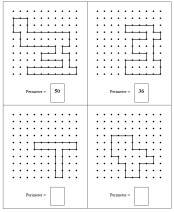Write the Perimeter - first row answers given (smaller shapes) Write the Perimeter - first row answers given (larger shapes) Write the Perimeter (smaller shapes) Write the Perimeter (larger shapes)

Rectangles Shown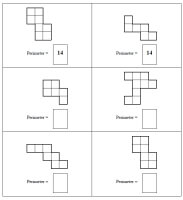Write the Perimeter - first row answers given (smaller shapes) Write the Perimeter - first row answers given (larger shapes) Write the Perimeter (smaller shapes) Write the Perimeter (larger shapes)

More Challenging - Indent Lines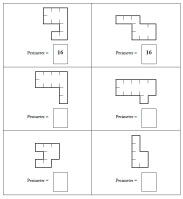Write the Perimeter - first row answers given (smaller shapes) Write the Perimeter - first row answers given (larger shapes) Write the Perimeter (smaller shapes) Write the Perimeter (larger shapes)

Most Challenging - Indent Lines (fewer indent lines given)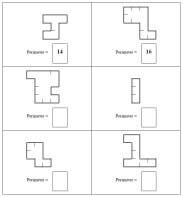Write the Perimeter - first row answers given (smaller shapes) Write the Perimeter - first row answers given (larger shapes) Write the Perimeter (smaller shapes) Write the Perimeter (larger shapes)

Rectangles Shown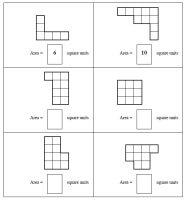Write the Area - first row answers given (smaller shapes) Write the Area - first row answers given (larger shapes) Write the Area (smaller shapes) Write the Area (larger shapes)

More Challenging - Indent Lines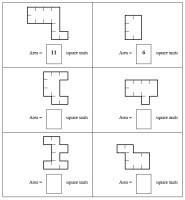Write the Area - first row answers given (smaller shapes) Write the Area - first row answers given (larger shapes) Write the Area (smaller shapes) Write the Area (larger shapes)

Most Challenging - Indent Lines (fewer indent lines given)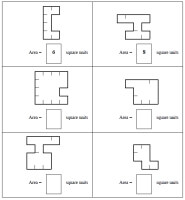Write the Area - first row answers given (smaller shapes) Write the Area - first row answers given (larger shapes) Write the Area (smaller shapes) Write the Area (larger shapes)

Find the perimeter of each figure
Find the perimeter of each figure using a ruler
Perimeter: Find the value of n
Find the area of each figure
Find the value of n
Find the area and perimeter of each rectangle (no graphics)
Find the perimeter of a complex polygon (composed of 2 rectangles)
Find the perimeter of a complex polygon (composed of 3 rectangles)
Find the perimeter of a complex polygon (composed of 4 rectangles)
Find the area and perimeter of a complex polygon (composed of 2 rectangles)
Find the area and perimeter of a complex polygon (composed of 3 rectangles)
Find the area and perimeter of a complex polygon (composed of 4 rectangles)
Area and Perimeter Mixed Review

Compare and Contrast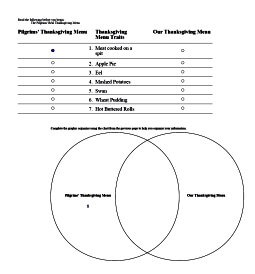Measurement Traits: Compare and contrast perimeter and area as units of measure. (Grades 3-4)

Find the perimeter of each figure
Find the perimeter of each figure using a ruler
Perimeter: Find the value of n
Find the area of each figure
Find the value of n
Find the area and perimeter of each rectangle (no graphics)
Find the perimeter of a complex polygon (composed of 2 rectangles)
Find the perimeter of a complex polygon (composed of 3 rectangles)
Find the perimeter of a complex polygon (composed of 4 rectangles)
Find the area and perimeter of a complex polygon (composed of 2 rectangles)
Find the area and perimeter of a complex polygon (composed of 3 rectangles)
Find the area and perimeter of a complex polygon (composed of 4 rectangles)
Area of a parallelogram (lengths are whole numbers)
Area of a parallelogram (lengths are decimals)
Area of a parallelogram (lengths are fractions)
Area of a triangle (lengths are whole numbers)
Area of a triangle (lengths are decimals)
Area of a triangle (lengths are fractions)
Find the circumference (graphics; lengths are whole numbers)
Find the circumference (graphics; lengths are decimals)
Find the circumference (graphics; lengths are fractions)
Find the circumference (lengths are whole numbers)
Find the circumference (lengths are decimals)
Find the circumference (lengths are fractions)
Area and Perimeter Mixed Review (Grade 5)

Find the perimeter of each figure
Find the perimeter of each figure using a ruler
Perimeter: Find the value of n
Find the area of each figure
Find the value of n
Find the area and perimeter of each rectangle (no graphics)
Find the perimeter of a complex polygon (composed of 2 rectangles)
Find the perimeter of a complex polygon (composed of 3 rectangles)
Find the perimeter of a complex polygon (composed of 4 rectangles)
Find the area and perimeter of a complex polygon (composed of 2 rectangles)
Find the area and perimeter of a complex polygon (composed of 3 rectangles)
Find the area and perimeter of a complex polygon (composed of 4 rectangles)
Area and Perimeter Mixed Review (Grade 4)

Part 2
Area of a parallelogram (lengths are whole numbers)
Area of a parallelogram (lengths are decimals)
Area of a parallelogram (lengths are fractions)
Area of a triangle (lengths are whole numbers)
Area of a triangle (lengths are decimals)
Area of a triangle (lengths are fractions)
Find the circumference (graphics; lengths are whole numbers)
Find the circumference (graphics; lengths are decimals)
Find the circumference (graphics; lengths are fractions)
Find the circumference (lengths are whole numbers)
Find the circumference (lengths are decimals)
Find the circumference (lengths are fractions)
Area and Perimeter Mixed Review (Grade 5)

Area and Circumference of a Circle
Perfect Squares and Square Roots
Write each perfect square, using the power 2
Write each perfect square as a square root
Find the square root
Find the value of n
Estimate a square root (multiple choice)
Circumference
Find the circumference (graphics; lengths are whole numbers)
Find the circumference (graphics; lengths are decimals)
Find the circumference (graphics; lengths are fractions)
Find the circumference (lengths are whole numbers)
Find the circumference (lengths are decimals)
Find the circumference (lengths are fractions)
Area of a Circle
Area of a circle (graphics; lengths are whole numbers)
Area of a circle (graphics; lengths are decimals)
Area of a circle (graphics; lengths are fractions)
Area of a circle (lengths are whole numbers)
Area of a circle (lengths are decimals)
Area of a circle (lengths are fractions)
Find the area of the partial circles
Review
Area and Circumference of a Circle Mixed Review

Perimeter, Circumference, and Area
Find area of triangles (given coordinates)
Find area of rectangles and squares (given coordinates)
Find area of trapezoids (given coordinates)
Find area review (given coordinates)
Area and perimeter word problems: rectangles and parallelograms
Area and perimeter: rectangles with fractions
Area and perimeter of rectangles (algebra)
Area and perimeter: triangles
Area and perimeter: trapezoids
Area and perimeter: triangles (with fractions)
Area and perimeter: trapezoids (with fractions)
Pythagorean theorem
Circles: Find the circumference
Circles: Find the area
Circles: Find the circumference and area
Perimeter and circumference - Word Problems Printable
Area - Word Problems Printable
Perimeter, Circumference, and Area Review Printable

Area and Circumference of a Circle (Grades 6-7)
Perfect Squares and Square Roots
Write each perfect square, using the power 2
Write each perfect square as a square root
Find the square root
Find the value of n
Estimate a square root (multiple choice)
Circumference
Find the circumference (graphics; lengths are whole numbers)
Find the circumference (graphics; lengths are decimals)
Find the circumference (graphics; lengths are fractions)
Find the circumference (lengths are whole numbers)
Find the circumference (lengths are decimals)
Find the circumference (lengths are fractions)
Area of a Circle
Area of a circle (graphics; lengths are whole numbers)
Area of a circle (graphics; lengths are decimals)
Area of a circle (graphics; lengths are fractions)
Area of a circle (lengths are whole numbers)
Area of a circle (lengths are decimals)
Area of a circle (lengths are fractions)
Find the area of the partial circles
Review
Area and Circumference of a Circle Mixed Review

Volume and Surface Area
Surface area and volume of right rectangular prisms
Volume of prisms and cylinders (Graphics)
Volume of cones, pyramids, and spheres (Graphics)
Volume of prisms, cylinders, cones, pyramids, and spheres
Surface area of prisms and cylinders
Surface area of pyramids, cones, and spheres
Volume Word Problems Printable
Volume and Surface Area Review Printable

Matrices Worksheets
Determinants: Calculate area of triangles

Conic Sections
Circles: Find the center, circumference, and area

Coordinate Geometry
Graph the Line Segment
Find Area of a Triangle [given 3 points]
Find Area of Trapezoids
Review of Find Area
Find the Slope of a Line [given 2 points]
Determine if 2 lines are Parallel
Write Equation for a Line [given m and b]
Find the slope and y-intercept from an Equation
Find an Equation [given m and a point on the line]
Find an Equation [given two points on a line]
Mixed Review: Find an Equation
Graphing Linear Equations [using x- and y- intercepts method]
Graphing Linear Equations [using slope intercept method]
Graphing Linear Equations [using any method]
Graphing Linear Equations [using any method]
Solve 2 Equations Graphically [solutions are small whole numbers]
Solve 2 Equations Algebraically
Using Subtraction to Find Area
Mixed: Find Area of Triangles, Parallelograms, Trapezoids, and Squares.
Find the Midpoint (Given 2 Points)
Find the Missing Point (Given a Midpoint and either A or B of Line AB)
Distance: Find the Distance Between Two Points
Distance: Between A and the Midpoint (Given Two Points)
Determine if points ABCD are a Quadrilateral.
Find the Slope and Length of a Line (Given Points A and B)
Determine Whether Lines are Parallel or Perpendicular
Given a Slope and Points, calculate the missing coordinate
Determine if the Points are Collinear
Given Collinear Points, Find Missing Coordinate
Slope of Parallel and Perpendicular Lines
Prove that a Triangle is a Right Triangle
Prove that a Quadrilateral is a Parallelogram
Prove that a Quadrilateral is a Rectangle
Prove that a Quadrilateral is a Square
Mixed from last 4 worksheets

Have a suggestion or would like to leave feedback?